Want to share your content on R-bloggers? click here if you have a blog, or here if you don't.

Yesterday evening, I discovered some interesting slides on False-Positives, p-Hacking, Statistical Power, and Evidential Value, via  ‘s post on Twitter. More precisely, there was this slide on how cheating (because that’s basically what it is) to get a ‘good’ model (by targeting the p-value)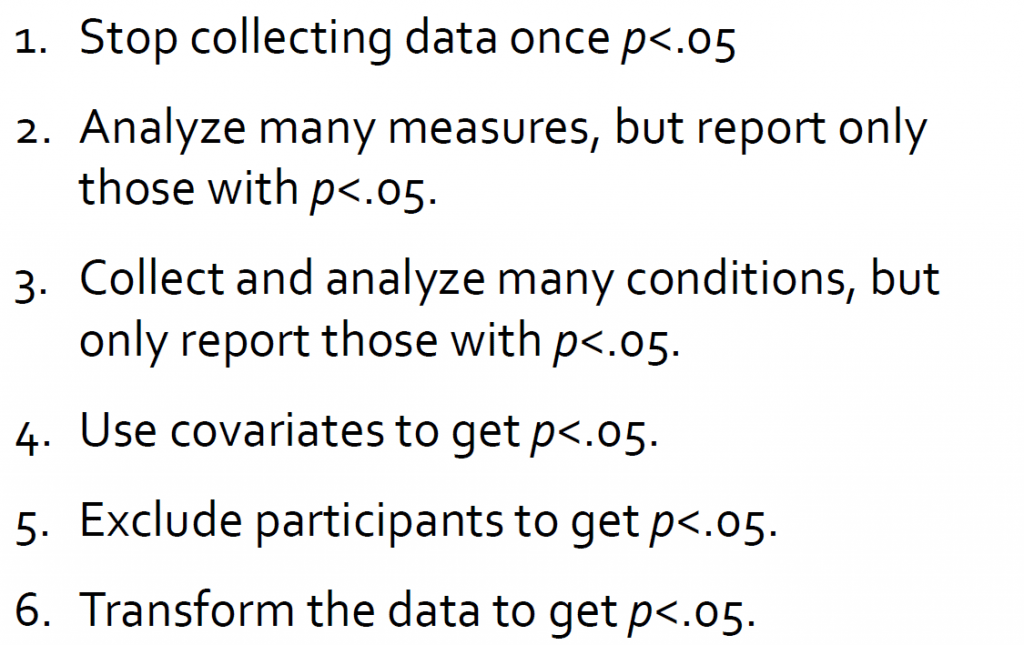As mentioned by @david_colquhoun  one should be careful when reading the slides : some statistician might have a heart attack when they read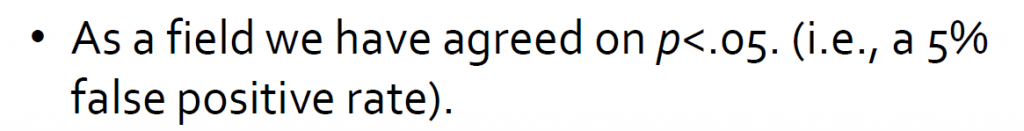But still, there are interesting points in that slide.

In Economics, there is an old saying: “when a measure become a target, it is no longer a measure“. That’s Goodhart’s law. Which is probably the most important thought I heard in the past 15 years.

Indeed, it possible to get anything you like when playing like that. For instance the first point. Consider some observations, and we want to get a Gaussian sample. But unfortunately, it is not. E.g. generate some Student random variables.

```> n=1000
> set.seed(1)
> X=rt(n,df=5)
```

If we use Anderson Darling test (we want to test normality here), we should reject the assumption that our sample is normaly distributed,

```> library(ADGofTest)
5.608129e-05
```

But it might be possible to select ‘optimaly’ the good number of observations we should keep

```> PV=function(n) ad.test(X[1:n],pnorm)\$p.value
> u=seq(121,500,by=5)
> v=Vectorize(PV)(u)
> plot(u,v,type="l",xlab="Sample Size")
> abline(h=.05,lty=2,col="red")```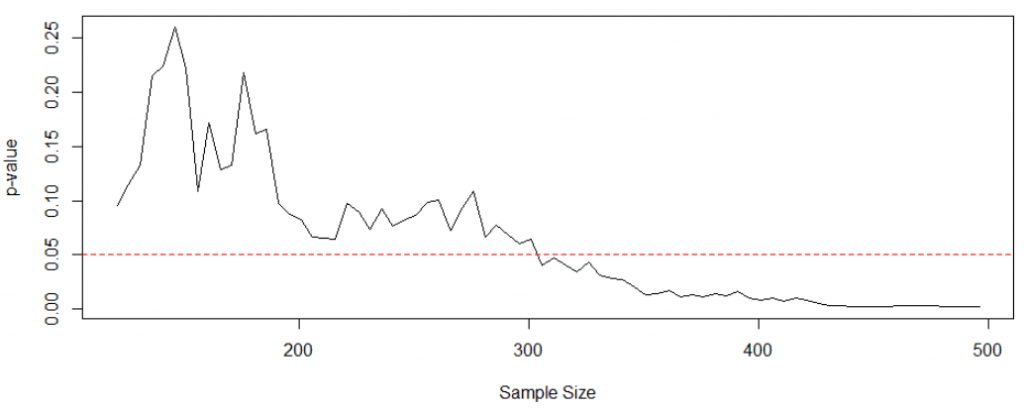Here, if we keep only the first 300 observations, we accept the Gaussian assumption. Actually, we standard statistical tests, the power is poor with a small number of observations. So basically, with a small number of observations, we should accept the null. But if the null is false, usually, with more observations, we reject it. That is the decreasing (more or less) trend that we oberve above, as a function of the sample size. So if we stop soon enough our study, it should be fine, we should accept the null.

An alternative, with randomized trials is to properly choose what ‘randomized’ means. For instance, assume that we must have 1,000 observations. Then use

```> seed=function(s){
+   set.seed(s)
+   X=rt(1000,df=5)
+ }
> test=FALSE
> s=1
> while(test==FALSE){test=seed(s); s=s+1}
> print(s-1)
 1201```

With that very specific seed, for our random sample, we should accept the null (even if it is not valid)

```> set.seed(1201)
> X=rt(1000,df=5)
0.08371768```

Last, but not least, a classical techniques that every one in politics knows about : if the measure is not fine, change the measure. Here, do not use Anderson Darling test, but use another one…. There are (so) many tests for normality.

```> library(normtest)
> mult_seed=function(s){
+   set.seed(s)
+   X=rt(200,df=5)
+   pv=c(ajb.norm.test(X)\$p.value,
+        frosini.norm.test(X)\$p.value,
+        jb.norm.test(X)\$p.value,
+        kurtosis.norm.test(X)\$p.value,
+        skewness.norm.test(X)\$p.value,
+        wb.norm.test(X)\$p.value
+        )
+   return(pv)
+ }```

Hence,

```> mult_seed(10)
 0.1055 0.6505 0.1295 0.0715 0.4295 0.1785```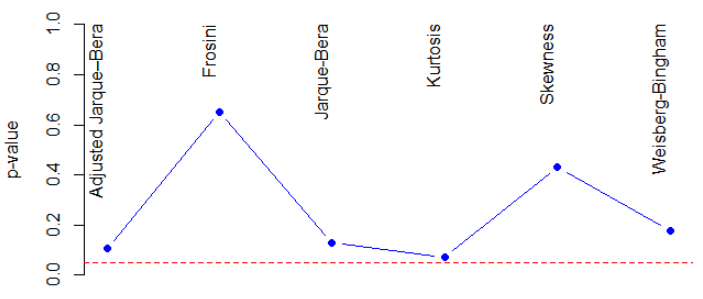or

```> mult_seed(53)
 0.0050 0.2455 0.0030 0.0005 0.9610 0.0105
```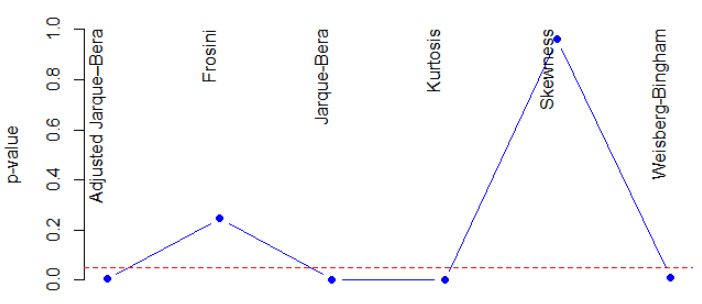Fun, isn’t it? But that clearly not how we should run a statistical analysis!